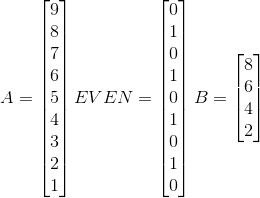# Indexing with ArrayFire

ArrayFire is a fantastic library when it comes to performance. One of the things that people overlook when looking into ArrayFire is it’s powerful indexing capabilities.

The main data structure in the ArrayFire library is the array. The array stores the data in a column major order. This means that the following code:

```float a[] = {1, 2, 3, 4, 5, 6, 7, 8, 9};
array A(3, 3, a);
```

Will produce the following matrix:Notice how the first three values of the `a` array make up the first column of `A`.

When you want to perform an operation on every element of the array you can just use the variable name with the operation. The following command adds 5 to each element of the array:

```A += 5;
```

If you want to assign one value to each element of the array, you will be tempted to do something like this:

```A = 3; // Don't do this
```

This operation will actually create an array with a single element with the value of 3. In order to perform this operation you will need to use the use the `constant` function or the span object(more on this later).

```A = constant(3, A.dims()); // Okay
A(span) = 3;               // Also works
```

Sometimes you only want to perform a calculation on a part of the data. In this case you can index into the elements of the matrix using the parenthesis notation. For example, you can access the middle element of the initial array by using:

```float a[] = {1, 2, 3, 4, 5, 6, 7, 8, 9};
array A(3, 3, a);
array B = A(1, 1);
// B == 5
```

The first number in the parenthesis is the column index, and the second number is the row index. You can index in this way to up to 4 dimensions.

ArrayFire also comes with a host of helper functions which allow you to access whole rows and columns of a matrix. The `row` and `col` functions will return an array which is made up of elements in the row an column respectively. You can access a set of rows and columns using the `rows` and `cols` functions.

```float a[] = {1, 2, 3, 4, 5, 6, 7, 8, 9};
array A(3, 3, a);
array B = A.row(2);
array C = A.cols(1,2);
```You can also use parenthesis notation to extract rows and columns of a matrix or volume. In order to do this, we will use the `span` and `seq` objects to specify which rows and columns we need.

The `seq` object returns a sequence of values. For example, you can create a sequence of `{0, 1, 2, 3}` by calling `seq(4)`. The `span` object is a sequence which gives all the elements of an array. Using these objects you can do some pretty sophisticated indexing. The previous example can be rewritten as the following:

```float[] a = {1, 2, 3, 4, 5, 6, 7, 8, 9};
array A(3, 3, a);
array B = A(2, span);
array C = A(span, seq(1,2));
```

The values of B and C should be identical to the first example.

You can even index into an array using another array object. The values of the array object passed into the parenthesis will be used as indices. Here is an example:

```float a[] = {9, 8, 7, 6, 5, 4, 3, 2, 1};
float idx[] = {1, 3, 5};
array A(9, a);
array IDX(3, idx);
array B = A(IDX); //B == {8, 6, 4}
```

We first create two C-style arrays. The first array contains the values from 9 to 1 and the second array contains the indices we want to access. We create array objects from these values and pass the `IDX` as the index of array `A`. The resulting array B will contain the values `{8, 6, 4}` which corresponds to the indices `{1, 3, 5}`.

If the passed array object is a b8(boolean) type, then only the non-zero values will be returned.

```float a[] =  {9, 8, 7, 6, 5, 4, 3, 2, 1};
bool  even[] =   {0, 1, 0, 1, 0, 1, 0, 1, 0};
array A(9, a);
array EVEN(9, even);
array B = A(EVEN);
```This technique allows you to input relation operators as indexes. For example, we can extract all values which are greater than 5 using the following code:

```float a[] =  {9, 8, 7, 6, 5, 4, 3, 2, 1};
array A(9, a);
array B = A(A > 5);
```I hope this blog was helpful for anyone who is interested in what ArrayFire has to offer. This is the first part of the indexing series. In a future post I will talk about more advanced topics including performance and memory accesses.